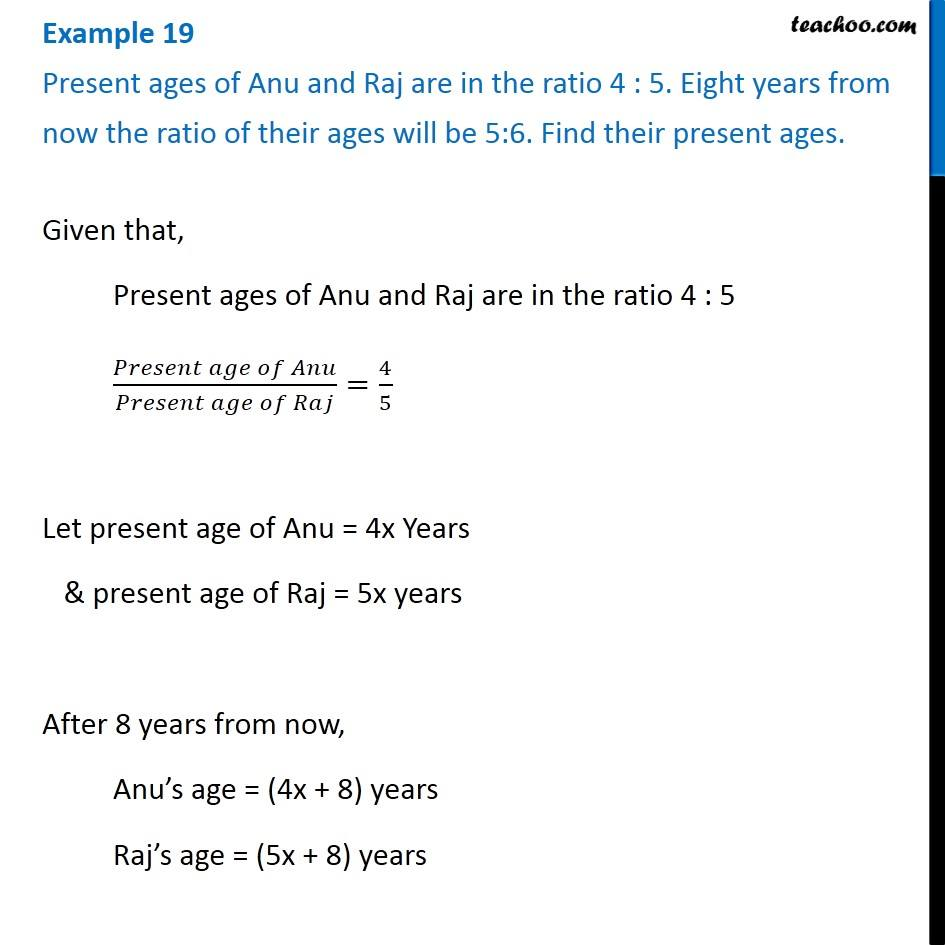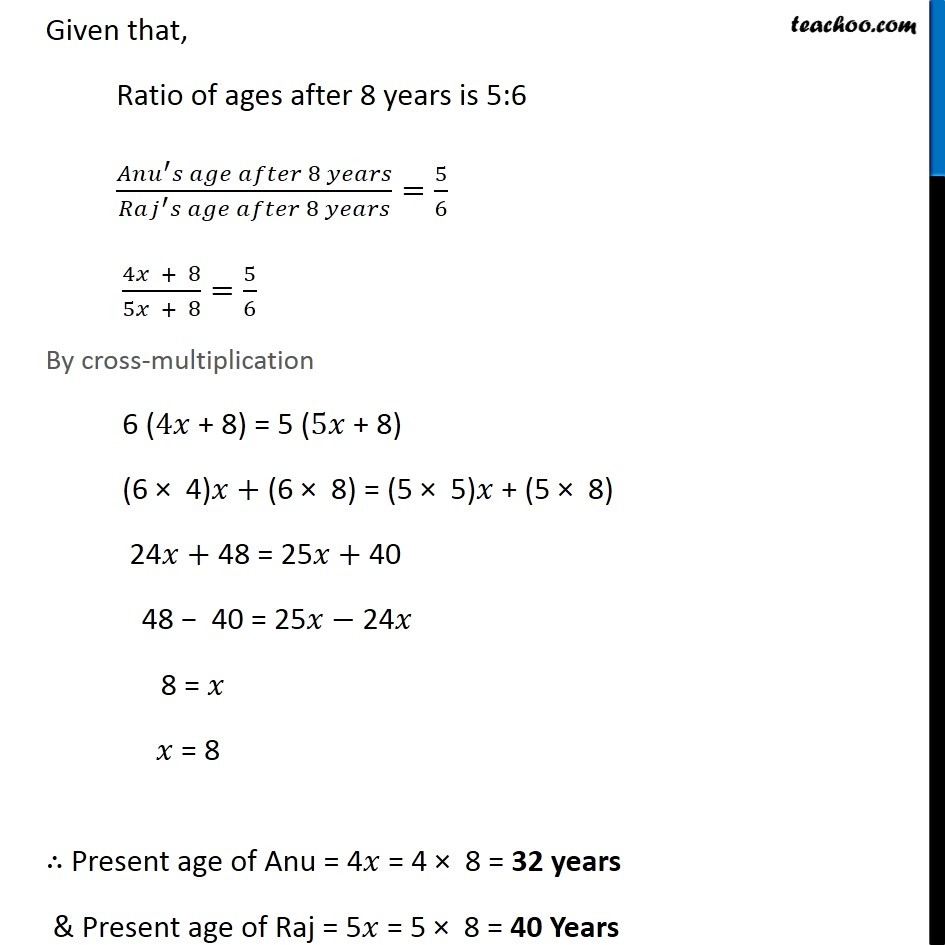Examples

Chapter 2 Class 8 Linear Equations in One Variable
Serial order wiseLearn in your speed, with individual attention - Teachoo Maths 1-on-1 Class

### Transcript

Question 15 Present ages of Anu and Raj are in the ratio 4 : 5. Eight years from now the ratio of their ages will be 5:6. Find their present ages. Given that, Present ages of Anu and Raj are in the ratio 4 : 5 (𝑃𝑟𝑒𝑠𝑒𝑛𝑡 𝑎𝑔𝑒 𝑜𝑓 𝐴𝑛𝑢)/(𝑃𝑟𝑒𝑠𝑒𝑛𝑡 𝑎𝑔𝑒 𝑜𝑓 𝑅𝑎𝑗)=4/5 Let present age of Anu = 4x Years & present age of Raj = 5x years After 8 years from now, Anu’s age = (4x + 8) years Raj’s age = (5x + 8) years Given that, Ratio of ages after 8 years is 5:6 (𝐴𝑛𝑢^′ 𝑠 𝑎𝑔𝑒 𝑎𝑓𝑡𝑒𝑟 8 𝑦𝑒𝑎𝑟𝑠)/(𝑅𝑎𝑗^′ 𝑠 𝑎𝑔𝑒 𝑎𝑓𝑡𝑒𝑟 8 𝑦𝑒𝑎𝑟𝑠)=5/6 (4𝑥 + 8)/(5𝑥 + 8)=5/6 By cross-multiplication 6 (4𝑥 + 8) = 5 (5𝑥 + 8) (6 × 4)𝑥+ (6 × 8) = (5 × 5)𝑥 + (5 × 8) 24𝑥+ 48 = 25𝑥+ 40 48 − 40 = 25𝑥− 24𝑥 8 = 𝑥 𝑥 = 8 ∴ Present age of Anu = 4𝑥 = 4 × 8 = 32 years & Present age of Raj = 5𝑥 = 5 × 8 = 40 Years